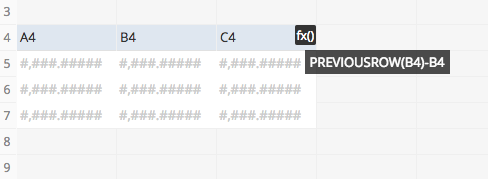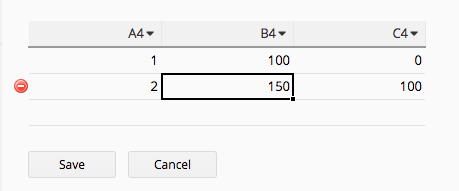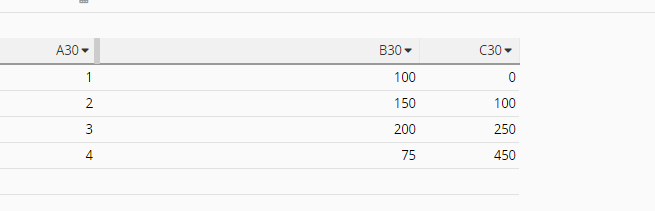# Formula Column - Cell Reference as opposed to Field Label Reference

I have a subtable on my form where each row hold various values relating to a specific year.

Each row (year) needs to house formula columns which reference and use values from the previous years record. They in turn being used as part of the calculation in next years values.

Is this possible?

Thanks

Hi Scott,

Would you mind to try if PREVIOUSROW(value) works for you?Hi Angie

I will give that a try!

Thank you

Hi Angie

That gives me the running balance for the column but I need to extract the previous rows value in that column.

Thanks

Hi Scott,

You can assign formula to minus that column from the retrieved running balance:Hi Amy

Thank you. I need to keep calculating the last columns value so in the above example I’d need C7 to show 150. Is that possible?

Hi Scott,

To retrieve last value of the column from subtable, you can use LAST() formulas.

Hi Amy

Sorry, my bad terminology. That should have said ‘previous’ not ‘last’. In your example I need to see the previous value for B in the current row. So every row that is added looks up the previous rows value for B.

Hi Scott,